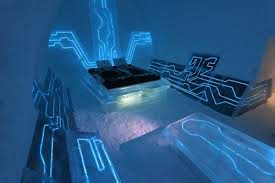Published on Sep 03, 2023

### Abstract

The Objective : If the smaller the holes on top of the tube would it differ from the size of the flames and the volume?

### Methods/Materials

If I drilled the holes 1/2inch from each other, then how about if I do it in a differ measurement will it change any part of the experiment? If I use a smaller tank of propane verses a bigger tank of propane will it make a difference to the size of flame that will be emitted from the tube? What if I want to measure the wavelengths of the soundwaves is it possible to measure it with the use of an algebraic equation to figure out the solution?

Ventilation Ducting Brackets x2 Propane Tubing Hose T Connector Hose Splicers x2 Latex Sheets Scrap Wood Drill Hammer Tape Measure Knife or Scissors Silicone Sealant Epoxy Putty Duct Tape Masking Tape Teflon Tape

### Results

1 The smaller the hole the less gas escapes the tube but there's more pressure inside the tube thus the emitted flame is more defined.The bigger the hole more gas is being let out so there's lesser pressure and less defined flame.

2 Spacing the holes 1/2inch apart made the flames more well defined and uniform than using hole 1/4 inch apart.

3 Another replica of another Ruben's Tube was made and had a smaller tank of propane it showed that there wasn't enough gas transmitting into the tube, making the flames not go any higher then 1/2inch thus a smaller tank of propane couldn't be more effective than a regular size tank.

4 The speed of sound is about 340 meters per second at sea level. Sound is a vibration so it would be measured by hertz (Hz) -the SI unit of frequency equal to one cycle per second. The frequency(f)=to the speed (v) of the wave then dividing it by the wavelength (lambda) of the wave(Frequency is = speed/wavelength f=v/lambda)

### Conclusions/Discussion

Now to find the wavelength I multiplied by lambda and divided it by (f) to get- Lambda= v/f To test this I used an example of 360 hertz and used the rough speed of sound as(v) Ex)lambda=340(m/s)/360 hertz. It gave me the value for lambda as 0.94meters

The Ruben's Tube is a physics experiment demonstrating standing waves and the relationship between air pressure and sound waves.